# Go Math Grade 3 Answer Key Chapter 12 Two-Dimensional Shapes Assessment Test

This chapter can improve student’s math skills, by referring to the Go Math Grade 3 Answer Key Chapter 12 Two-Dimensional Shapes Assessment Test, and with the help of this Go Math Grade 3 Assessment Test Answer Key, students can score good marks in the examination

Go Math Grade 3 Answer Key Chapter 12 contains all the topics of chapter 12 which helps to test the student’s knowledge. Through this assessment test, students can check their knowledge. This assessment test is also helpful for the teachers to know how much a student understood the topics.

Chapter 12:

### Test – Page 1 – Page No. 121

Question 1.
Which words describe this shape? Mark all that apply.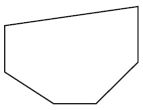Options:
a. polygon
b. hexagon
c. open shape

Explanation: In geometry, a polygon is a plane figure that is described by a finite number of straight-line segments connected to form a closed polygonal chain or polygonal circuit.

Question 2.
Sam drew one side of a rhombus with at least 1 right angle. Draw the other 3 sides to complete Sam’s shape.Explanation: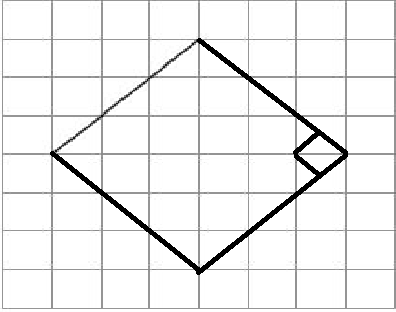A rhombus is a Parallelogram with 4 equal sides. Because it is a parallelogram, opposite angles are congruent. And because it is a quadrilateral the sum of all angles must be equal to 360 degrees. So, if one angle is right then the opposite angle is 90 degrees. Then the 1st pair has a sum of 180 degrees, then 360–180 = 180, so the other pair of angles sum to 180 degrees. But the other pair of angles are also opposite of each other so must be congruent, so each of the other two angles is also 90 degrees. So, if one angle is right all 4 angles are right angles. These kinds of rhombi, or rhombuses, are called squares. Squares are a subset of rhombi.
So after completing the shape with the other three sides we get a Square.

Question 3.
Louie has a stamp with this shape.For numbers 3a–3d, select True or False for each statement about the shape.
a. The shape has no right angles.
i. True
ii. False

Explanation: Pentagon can have right angles too. It can have a maximum of 3 right angles but here the given diagram seems like a regular Pentagon, which is having each interior angle of a regular pentagon = 108 degrees. Thus there is no right angle.

Question 3.
b. The shape has 5 angles greater than a right angle.
i. True
ii. False

Explanation: The sum of the interior angles should be equal to 540°. Since here in the current pentagon, there is no right angle if we divide 540/5 minimum angle would be 108°. Hence the shape has 5 angles greater than a right angle.

Question 3.
c. The shape has 2 angles less than a right angle.
i. True
ii. False

Explanation: Given shape is a regular pentagon which is having a regular interior angle of 108° each.

Question 3.
d. The shape has 1 right angle.
i. True
ii. False

Explanation: Given shape is a regular pentagon which is having a regular interior angle of 108° each.

### Test – Page 2 – Page No. 122

Question 4.
Jane used a Venn diagram to sort shapes.Part A
Draw a plane shape that belongs inside the overlap region of the diagram.

Explanation: Overlap region has Quadrilateral/Polygons having right angles which are Square and rectangle.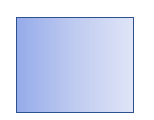Question 4.
Part B
How can you describe the shapes that belong in the section where the circles overlap?

Answer: To understand clearly the intersection part first we have to understand Polygon with the right angle and Quadrilaterals.

Explanation:
Polygon: In geometry, a polygon is a plane figure that is described by a finite number of straight-line segments connected to form a closed polygonal chain or polygonal circuit. The solid plane region, the bounding circuit, or the two together, maybe called a polygon

Right-angled Polygon: A polygon whose interior angles are 90°.

Quadrilaterals: In geometry, a quadrilateral is a polygon with four edges and four vertices.

So here in the given diagram intersection part comes under a Polygon/Quadrilateral having one or more interior angles

Question 5.
Write one of the following words to name each part of the figure.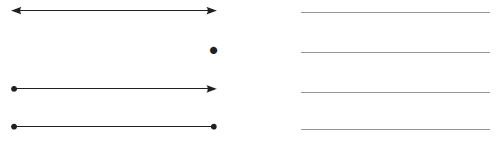Question 6.
Describe the angles and sides of this triangle.Answer: All triangles have interior angles adding to 180°. When one of those interior angles is 90°, it is a right angle and the triangle is known as the right triangle. In drawing the interior 90° angle is indicated with a small square in the vertex. The side opposite to the right angle is its hypotenuse, which is the longest side of all three. The other two angles in a right triangle add to 90°, they are complementary. The sides opposite to the complementary angles are the triangle’s legs.

### Test – Page 3 – Page No. 123

Question 7.
Which words describe this shape? Mark all that apply.Options:
a. rectangle
b. rhombus
d. square

Explanation:
Quadrilaterals: In geometry, a quadrilateral is a polygon with four edges and four vertices.
Rhombus: A rhombus is a quadrilateral whose four sides all have the same length.
The given figure is having 4 edges and 4 vertices that come under Quadrilateral also seem like having all the four sides of the same length which comes under rhombus too.

Question 8.
Divide each shape into the number of equal parts shown.
Then write the fraction that describes each part of the whole.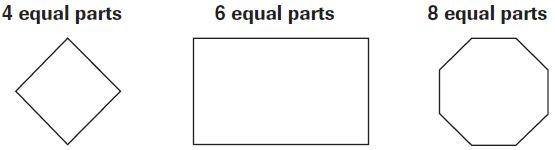Explanation: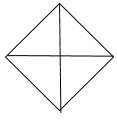The given shape is dived into 4 equal parts. Therefore, the fraction of each part of the whole is ¼ (one fourth).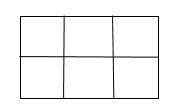The given shape has been divided into 6 equal parts. Therefore, the fraction of each part of the whole is 1/6.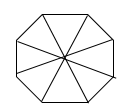The given shape has been divided into eight equal parts. Therefore, the fraction of each part of the whole is 1/8.

Question 9.
Cho drew a triangle with 3 angles that are each less than a right angle.
For numbers 9a–9d, choose Yes or No to tell whether that triangle could be the triangle Cho drew.
a.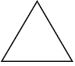i. yes
ii. no

Explanation: Cho drew a triangle having all the angles less than 90 degrees. So it could be Cho’s triangle.

Question 9.
b.i. yes
ii. no

Explanation: This triangle is having a right angle (90 degrees). So it could not Cho’s triangle.

Question 9.
c.i. yes
ii. no

Explanation: Tt is possible to be triangle drew by Cho. Since it is not having an angle which is a greater right angle.

Question 9.
d.i. yes
ii. no

Explanation: It could be Cho’s triangle too as it is not having an angle which is a greater right angle.

### Test – Page 4 – Page No. 124

Question 10.
Look at this group of shapes.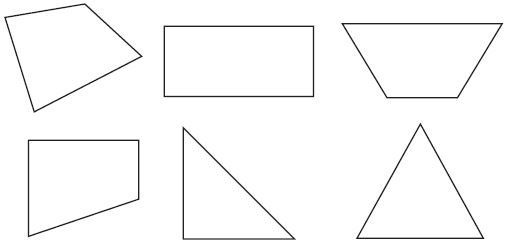Part A
Sort the shapes by sides. How many groups did you make? Explain how you sorted the shapes.

Explanation: We can sort by using the number of sides. The first group with two triangles having three sides each, Second group with Quadrilaterals having four edges and four vertices.

Question 10.
Part B
Sort the shapes by angles. How many groups did you make? Explain how you sorted the shapes.

Explanation: Sorted the shapes by angles, Right-angled polygons, and non-right angled polygons.

Question 11.
Gina drew a quadrilateral that always has 4 sides of equal length and 4 right angles. What quadrilateral did she draw?

Explanation: Square is the quadrilateral having 4 sides of equal length with 4 right interior angles.### Test – Page 5 – Page No. 125

Question 12.
Lacy used a Venn diagram to sort shapes. What label could she use for circle A?Explanation: Polygons with all sides of equal length.

Question 13.
Victor drew lines to divide a trapezoid into equal parts that represent $$\frac{1}{3}$$ of the whole area. Draw lines to show how Victor divided the trapezoid.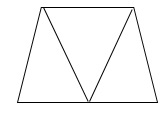Question 14.
Luisa drew this hexagon. Select the pairs of sides that appear to be parallel. Mark all that apply.Options:
a. a and b
b. b and e
c. c and f
d. d and a

Explanation: b and e, d and a are the pairs of sides that appear to be parallel.

### Test – Page 6 – Page No. 126

Question 15.
Tell why this shape is NOT a polygon.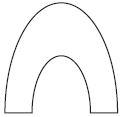Answer: A polygon by definition has straight line segments as its sides. If a geometrical figure has curved sides, it is not considered a polygon. Thus the given shape is not a Polygon.

Question 16.
Paige drew the triangle below. What statements describe the angles? Mark all that apply.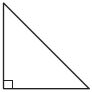Options:
a. At least one is less than a right angle.
b. One is a right angle.
c. All are less than a right angle.
d. One is greater than a right angle.

Explanation: Since one angle is the right angle other two angles are less than the right angle.

Question 17.
Bella drew a quadrilateral with 2 pairs of opposite sides that are parallel. The shape has no right angles and has sides that are of equal length. Draw a shape Bella could have drawn.

Answer: Could be a parallelogram as it will have parallel opposite sides without any right angle.

Explanation: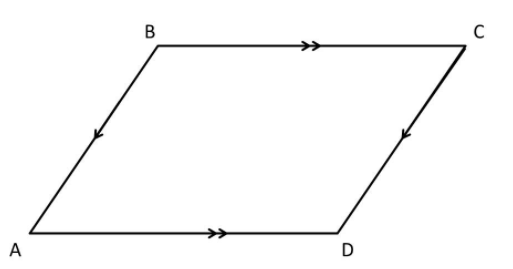Question 18.
For 18a–18d, select True or False for each description of a line segment.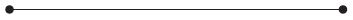a. straight
i. True
ii. False

Explanation: This is known as a line as it is an infinitely thin, infinitely long collection of points extending in two opposite directions

Question 18.
For 18a–18d, select True or False for each description of a line segment.
b. has 2 endpoints
i. True
ii. False

Explanation: It is having two endpoints.

Question 18.
c. continues in 1 direction
i. True
ii. False

Explanation: Line segment continue forever in zero directions.

Question 18.
d. part of a line
i. True
ii. False